Next: Bernoulli's Theorem Up: One-Dimensional Compressible Inviscid Flow Previous: Isentropic Flow

# Sound Waves

Suppose that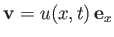,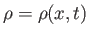,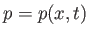, and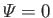in Equations (14.30) and (14.31). We obtain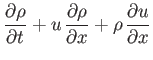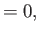(14.34)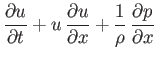(14.35)

Equation (14.32) implies that the flow is isentropic. In other words,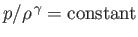along streamlines. Thus, it follows that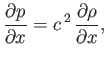(14.36)

where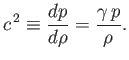(14.37)

Hence, Equations (14.34) and (14.35) become(14.38)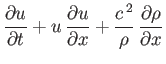(14.39)

Unfortunately, these equations are difficult to solve exactly, because of the presence of nonlinear terms such as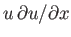. (See Example ix.)

Let us write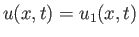,, and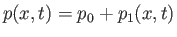, where quantities with the subscriptare assumed to be much smaller that corresponding quantities with the subscript 0 . Thus, we are now considering the evolution of small-amplitude pressure and density perturbations in a stationary gas of uniform density and pressure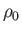and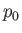, respectively. To first order in small quantities, the previous two equations yield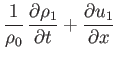(14.40)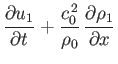(14.41)

where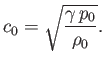(14.42)

The general solution to Equations (14.40) and (14.41) is well known (Fitzpatrick 2013):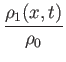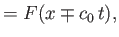(14.43)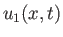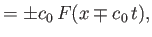(14.44)

where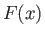is an arbitrary function. According to this solution, a small-amplitude density perturbation of arbitrary shape propagates through the gas, either in the positive or negative-direction (corresponding to the upper and lower signs, respectively), without changing shape, at the constant speed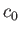. This type of perturbation is known as a sound wave, andis consequently referred to as the sound speed.

It is clear, from the previous analysis, that the general expression for the local sound speed in an isentropic ideal gas of pressure, density, and temperature, is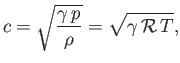(14.45)

where use has been made of Equation (14.1). It follows that the speed of sound is solely determined by the temperature of an ideal gas.

Let us now consider the effect of finite wave amplitude on the propagation of a sound wave through an isentropic ideal gas. The previous analysis suggest that, in a frame of reference moving with the local flow velocity,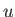, a sound wave propagates at the speed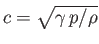. Thus, the net wave propagation speed is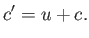(14.46)

Using the isentropic law,, to eliminatefrom, we deduce that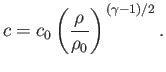(14.47)

Now, Equations (14.43) and (14.44) suggest that, in the presence of a sound wave propagating in the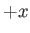-direction, the general differential relationship between the local flow velocity and the density is(14.48)

Making use of Equation (14.47), we can integrate the previous expression to give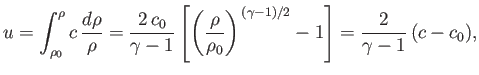(14.49)

or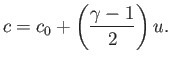(14.50)

It follows that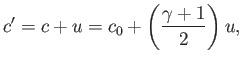(14.51)

or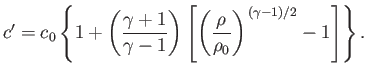(14.52)

Thus, we conclude that the net wave propagation speed is higher thanin regions of compression (i.e.,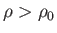), and lower in regions of rarefaction (i.e.,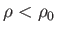). This implies that a finite-amplitude sound wave distorts, as it propagates, in such a manner that the regions of compression tend to catch up with the regions of rarefaction, leading to a monotonic increase in time of the velocity and temperature gradients in the former regions, and a monotonic decrease in the latter regions. Eventually, the velocity and temperature gradients in the regions of compression become so large that it is no longer valid to neglect the effects of viscosity and heat conduction, leading to a local breakdown of the isentropic gas law. Viscosity and heat conduction prevent any further steepening of the velocity and temperature gradients in the regions of compression, and the wave subsequently propagates without additional distortion. However, it has now effectively been transformed into a shock wave. (See Section 14.8.)

It is clear, from the previous discussion, that, at finite amplitude, there is an important difference in the behavior of compression and rarefaction waves propagating through a gas. A compression wave tends to steepen (i.e., the temperature and velocity gradients tend to increase), and eventually becomes a shock wave, in which case it is no longer isentropic. A rarefaction wave, on the other hand, always remains isentropic, because it tends to flatten, thereby, further reducing the velocity and temperature gradients. Hence, there are no rarefaction, or expansion, shocks.Next: Bernoulli's Theorem Up: One-Dimensional Compressible Inviscid Flow Previous: Isentropic Flow
Richard Fitzpatrick 2016-03-31